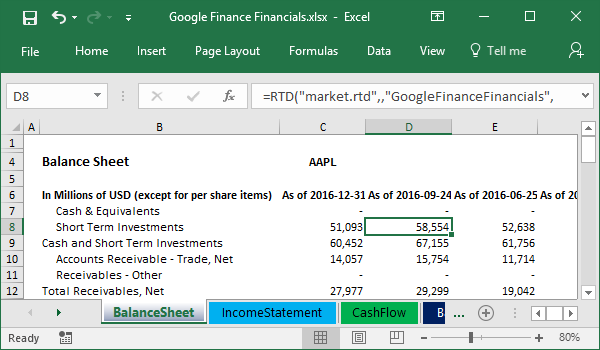MARKET.RTD Formula Builder

# MARKET.RTD Formula Builder

MARKET.RTD Formula Builder builds RTD formulas for the specified provider.

You can start it using Start, All Programs, MARKET.RTD, Formula Builder.

Formula Builder looks like this: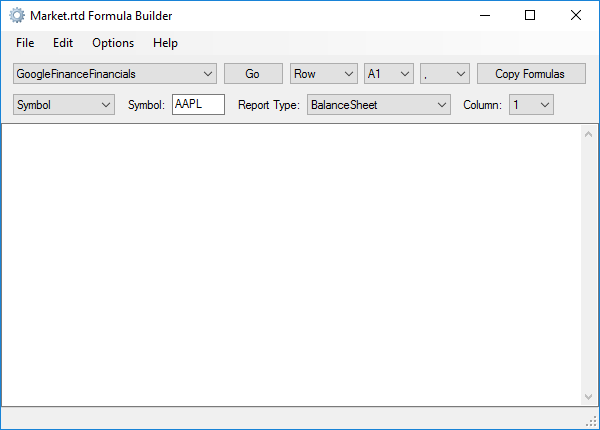First of all, select a provider: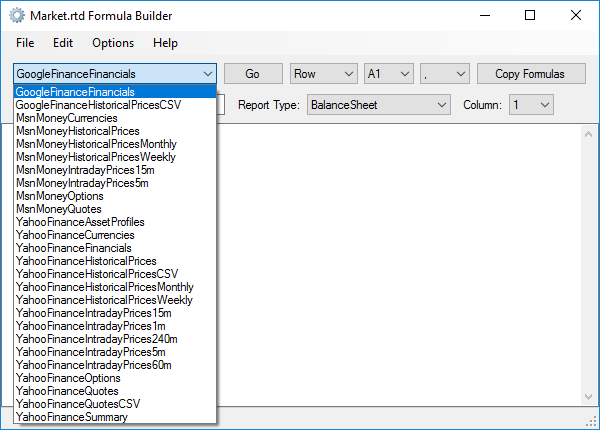Then select parameters in the second row and click the Go button. You will see the formulas like this: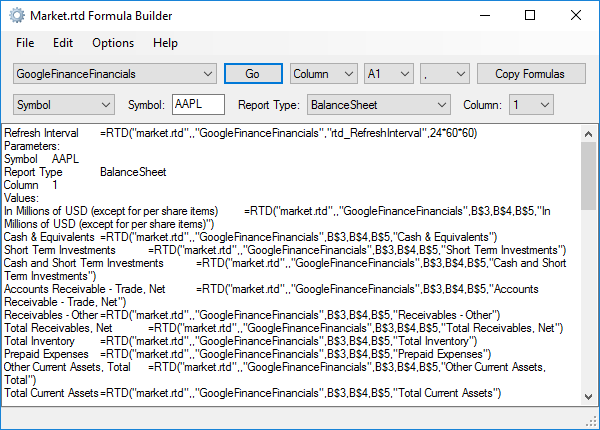You can customize the formula layout (Row, Column, or Absolute), formula style (A1 or RC) and the formula separator (comma or semicolon).

Then click Copy Formulas, create a new worksheet and paste formulas at cell A1.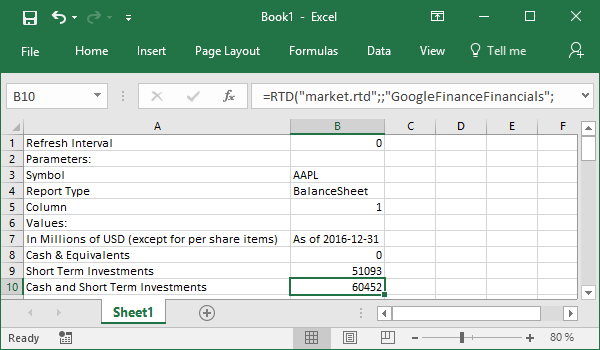Then you can format a worksheet as you need or copy formulas to your reports.

Note about formula parameters. In the example above, formulas use parameter values from cells B3-B5.

So, you can copy columns and change stock tickers or reports columns.

For example, the formatted report can look like this: